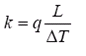# Thermal Resistance & Thermal Conductance

## Introduction

Thermal resistance (R) and thermal conductance (C) of the materials are reciprocals of one another and can be derived from thermal conductivity (k) and the thickness of the materials. The C-Therm TCi™ measures thermal conductivity and, therefore, paves the way to determine thermal resistance and thermal conductance.

### k-value – Thermal Conductivity

Thermal conductivity is the time rate of steady state heat flow through a unit area of a homogeneous material induced by a unit temperature gradient in a direction perpendicular to that unit area, W/m⋅K.

#### (1)Where,
L – Thickness of the specimen (m)
T – Temperature (K)
q – Heat flow rate (W/m2)

### R-value – Thermal Resistance

Thermal resistance is the temperature difference, at steady state, between two defined surfaces of a material or construction that induces a unit heat flow rate through a unit area, K⋅m2/W. According to this definition and Equation 1, Equation 2, therefore, can be obtained.

As indicated in Equation 2, the value of the thermal resistance can be determined by dividing the thickness with thermal conductivity of the specimen.

#### (2)### C-value – Thermal Conductance

Thermal conductance is the time rate of steady state heat flow through a unit area of a material or construction induced by a unit temperature difference between the body surfaces, in W/m2⋅K. C-value, hence, is the reciprocal of the R-value and can be expressed as Equation (3).

#### (3)Consequently, the value of the thermal conductance can be calculated by dividing the thermal conductivity with the thickness of the specimen.

## Conclusions

Thermal resistance and thermal conductance can be conveniently calculated from the thermal conductivity and thickness of the material. The C-Therm TCi™ is a flexible, rapid, non-destructive, highly sensitive and cost effective instrument that can directly measure thermal conductivity and thermal effusivity of a wide variety of samples, easing the process of determining thermal resistance and thermal conductance. For more informaiton on the TCi please contact us.